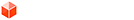## [Code] realign UVs, or: convert projective to affine

### [Code] realign UVs, or: convert projective to affine

When we want to work with textured organic meshes (like me in my current project), we want that the texture flows continuously over the surface. However it can happen that they become misaligned by editing etc.

I wrote this method and surprisingly figured out that the resulting mesh not only has an affine distortion but is still always continuous/seamless – even if it resulted from a projective distortion. That's something I've wanted for long for cases that don't work for projected textures.
projective2affine.gif

Of course the conversion is not lossless, the more subdivided the mesh is, the less noticeable is the difference.
Code: Select all
`def realign_uvs(entities=Sketchup.active_model.selection.to_a)  model = Sketchup.active_model   if Sketchup.version.to_i >= 7      model.start_operation("Repair misaligned UV coordinates", true)   else      model.start_operation("Repair misaligned UV coordinates")   end  @tw = Sketchup.create_texture_writer  side = true # frontside  hash = {} # vertex => [uvs]  faces = entities.find_all{|e| e.is_a?(Sketchup::Face) && (mat = (side)? e.material : e.back_material) && mat.materialType>0}  # 1) Let's collect all uvs at each vertex  faces.each{|f|    uv_helper = f.get_UVHelper(true, true, @tw)    f.vertices.each{|v|      p = v.position      uv = (side)? uv_helper.get_front_UVQ(p) : uv_helper.get_back_UVQ(p)      uv.x /= uv.z; uv.y /= uv.z; uv.z = 1      hash[v] = [] if !hash[v]      hash[v] << uv    }  }  # 2) Find the uv with the least aberration. Alternative: Calculate the average of the uvs at a vertex.  hash.each{|v, array|    #average_uv = array.inject(Geom::Point3d.new([0,0,0])){|sum, uv| sum+uv.to_a}.to_a.collect{|c| c/array.length.to_f}    #hash[v] = average_uv    best_uv = array.min{|uv_a,uv_b|      dist_a = array.inject(0){|dist, uv| d = uv_a.distance(uv); [d,dist].min} # sum of distances from uv_a      dist_b = array.inject(0){|dist, uv| d = uv_b.distance(uv); [d,dist].min} # sum of distances from uv_b      dist_b <=> dist_a}    hash[v] = best_uv  }  # 3) Apply the uvs for each face  faces.each{|f|    pt_array = []    vs = f.vertices[0..3]    vs.each{|v|      pt_array << v.position      pt_array << hash[v]    }    f.position_material(((side)? f.material : f.back_material), pt_array, side)  }  model.commit_operationend`
0
Last edited by Aerilius on Sat Dec 22, 2012 11:36 pm, edited 1 time in total.

Aerilius
PluginStore Author### Re: [Code] realign UVs, or: convert projective to affine

Looks very interesting. Though, could you explain "projective to affine" in this context? I'd like to understand this.
0
Thomas Thomassen — SketchUp Monkey & Coding addictthomthom
PluginStore Author### Re: [Code] realign UVs, or: convert projective to affine

Projective: like projected textures (all four texture pins modified) (this it what the TextureWriter makes unique when exporting)
Affine: skewed or scaled or stretched like a parallelogram, preserves parallel lines and equispaced points along lines.
(I find this document very helpful http://www.cs.cmu.edu/~ph/texfund/texfund.pdf p.13)
0

Aerilius
PluginStore Author